Home   Best PowerPoint Templates   Animated Math Equations For PowerPoint Presentations

## Animated Math Equations For PowerPoint Presentations

Math equations might be required in PowerPoint presentations to present complex calculations before an audience. For example, this might include a class lecture from a math or statistics teacher who might require revealing various formulas and calculations in the form of PowerPoint slides. The Animated Math Equations Template for PowerPoint provides a wide range of animated slides and equations which can be used for making slide decks with mathematical and geometry related icons and symbols.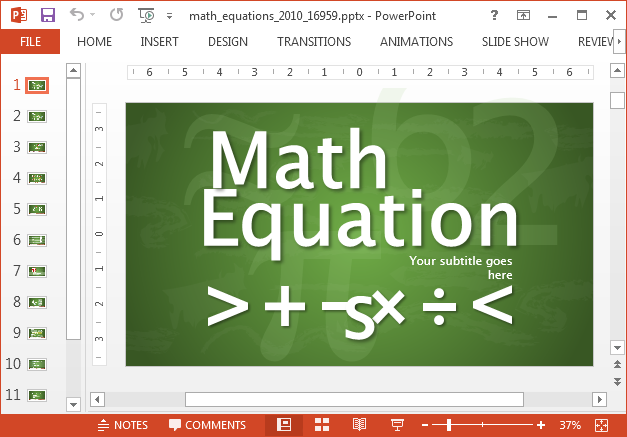### Editable Equations and Animated Layouts

With editable math equations and customizable layouts with animations, you get a very handy toolkit which can be easily moulded for different types of math and geometry related presentations. You can switch between animated and static layouts, as well as move objects around using nothing more than drag and drop.

Related: Free Math PowerPoint Templates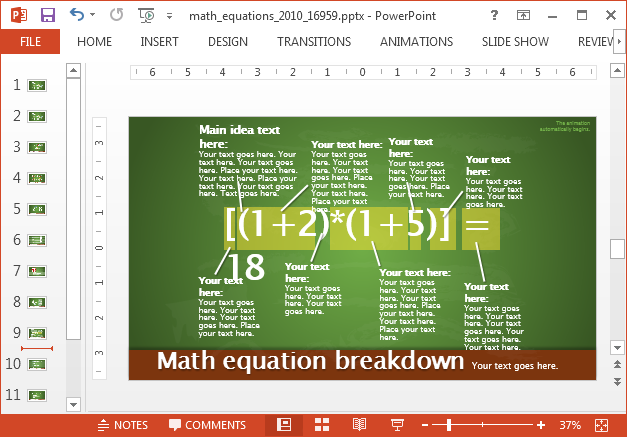### Highlight Parts of Your Mathematical Equations

There are also red circles which come within sample slides which can be moved over an equation to highlight parts of your slide. You can also copy these red circles to create more of them and even change the color of the circles.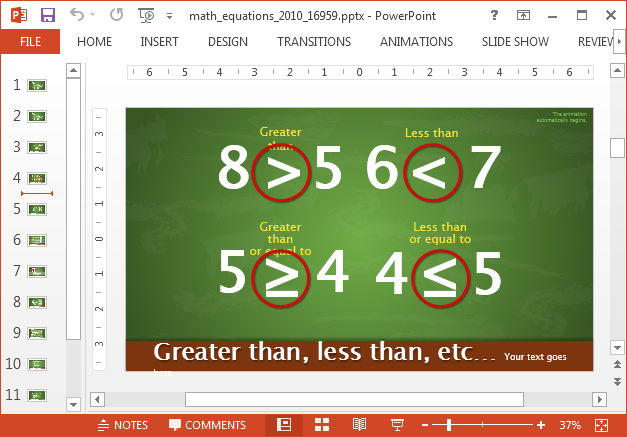### Create Complex Formulas and Equations in Slides

You can mix and mash the given equations to depict complex formulas and equations for your audience. The equations in sample slides act like clipart, which can be moved around, resized and recolored.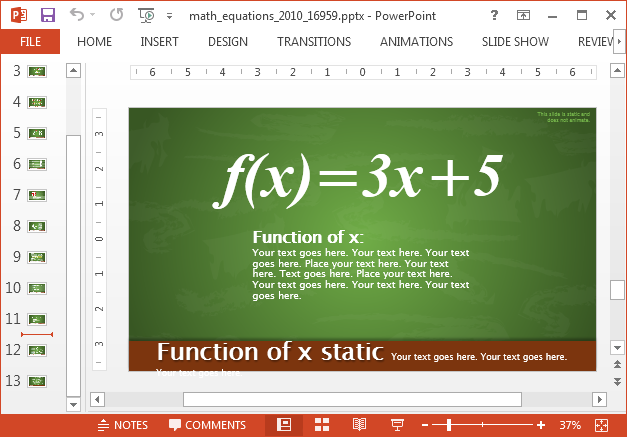### Sample Symbols and Clipart

There are also geometry symbols which can be incorporated in your slides. Moreover, the template also offers exclusive clipart slide which provides various useful symbols in the form of reusable clipart.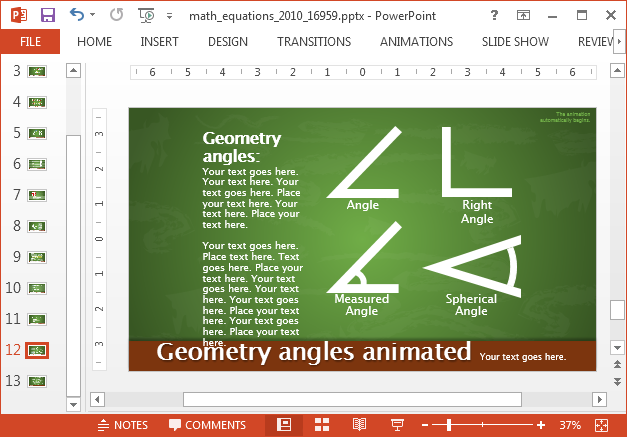The slide title ‘Useful Artwork’ is the last slide in the template and contains all major mathematical symbols. These symbols can help create equations, formulas and to explain basic or complex mathematical concepts.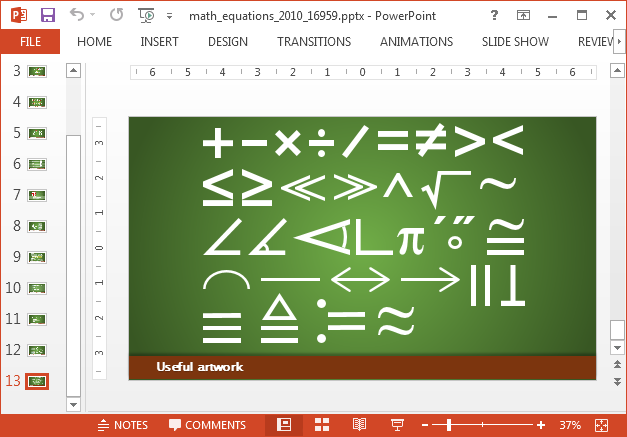Other than PowerPoint, both the Standard and Widescreen edition of this template are also available for Keynote.

Go to Presenter Media – Animated Math Equations Template for PowerPoint (Standard 4:3)

Go to Presenter Media – Animated Math Equations Template for PowerPoint (Widescreen 16:9)